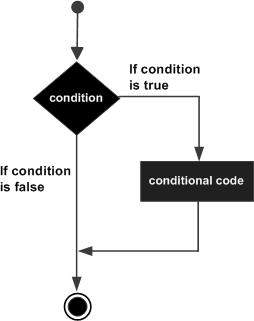# Go - if statement

An if statement consists of a boolean expression followed by one or more statements.

## Syntax

The syntax of an if statement in Go programming language is −

```if(boolean_expression) {
/* statement(s) will execute if the boolean expression is true */
}
```

If the boolean expression evaluates to true, then the block of code inside the if statement is executed. If boolean expression evaluates to false, then the first set of code after the end of the if statement (after the closing curly brace) is executed.

## Flow Diagram## Example

```package main

import "fmt"

func main() {
/* local variable definition */
var a int = 10

/* check the boolean condition using if statement */
if( a < 20 ) {
/* if condition is true then print the following */
fmt.Printf("a is less than 20\n" )
}
fmt.Printf("value of a is : %d\n", a)
}
```

When the above code is compiled and executed, it produces the following result −

```a is less than 20;
value of a is : 10
```
go_decision_making.htm